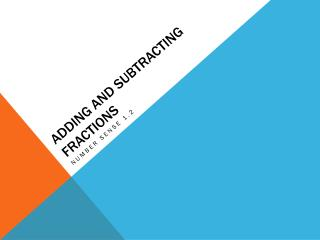DownloadDownload PresentationDownload Presentation- - - - - - - - - - - - - - - - - - - - - - - - - - - E N D - - - - - - - - - - - - - - - - - - - - - - - - - - -
##### Presentation Transcript

1. Adding and Subtracting Fractions Number Sense 1.2

2. In order to add/subtract fractions, the denominator must be the same. Example: ¼ + 2/4 = ¾ If the denominator is not the same, you must find the least common multiple of the denominators (This can also be called the Least Common Denominator). • How to add/subtract fractions.

3. 3/8 + 1/6 = [8 6] 4 3 2  4 3 LCM = 24 • Unlike Denominators: • Step 1: • Use the upside down cake method to determine the LCM • Step 2: The LCM becomes the new denominator for each fraction. 2

4. Step 3: • Determine what factor multiplies to make the LCM. Multiply the numerator by that factor. • Step 4: Always simplify when possible

5. 2/3 + 1/5 • Four Square Method: • Write the steps in you own words. • 1) • 2) • 3) • 4) 1 5 2 Denominator 3 Answer: 13/15

6. Practice Add. Answers: 1.) 13/15 2.) 7/9 3.) 16/21 4.) 7/8 5.) 5/8 • 1.) 2/3 + 1/5 • 2.) 4/9 + 1/3 • 3.) 3/7 + 1/3 • 4.) 1/2 + 3/8 • 5.) 3/8 + 1/4